• 特征函数和 cdf （cumulative distribution function，也叫分布函数）一样提供了另外一种描述随机变量的方法，φX(t)=E[eitX] \varphi_X(t) = \operatorname{E} \left [ e^{itX} \right ] 和 cdf 一样，能够完整地...
特征函数和 cdf （cumulative distribution function，也叫分布函数）一样提供了另外一种描述随机变量的方法，

φX(t)=E[eitX]<!--//--><![CDATA[//><!--
\varphi_X(t) = \operatorname{E} \left [ e^{itX} \right ]
//--><!]]>和 cdf 一样，能够完整地确定随机变量概率分布的性质。
展开全文• 概率论基础+复旦+李贤平版+课后答案+第四章数字特征与特征函数（1-14题）.doc
• UA MATH563 概率论的数学基础 中心极限定理24 随机变量的特征函数 定义 假设XXX是定义在(Ω,F,P)(\Omega,\mathcal{F},P)(Ω,F,P)上的随机变量，定义 ϕ(t)=E[eitX]\phi(t) = E[e^{itX}]ϕ(t)=E[eitX] 为XXX的特征...
UA MATH563 概率论的数学基础 中心极限定理24 随机变量的特征函数
定义 假设$X$是定义在$(\Omega,\mathcal{F},P)$上的随机变量，定义
$\phi(t) = E[e^{itX}]$
为$X$的特征函数(characteristic function)。
说明
记$\mu_X$为$X$的分布，则$\phi(t) = E[e^{itX}] = \int e^{itX}d\mu_X$
也就是说$\phi(t)$其实是$\mu_X$的Fourier变换，因此任意随机变量的特征函数总是存在的。我们可以将特征函数与矩母函数(moment generating function，也就是$\mu_X$的Laplace变换)做个对比，
$M_X(t) = E[e^{tX}]$
被称为矩母函数，当且仅当$E[e^{tX}]<\infty$时，矩母函数存在。而$|e^{itX}| \le 1$，因此$E[e^{itX}]$必定存在。
常用分布的特征函数

正态分布$N(\mu,\sigma^2)$:$\phi(t)=\exp(it\mu-\frac{\sigma^2t^2}{2})$
Gamma分布$\Gamma(\alpha,\beta)$:$\phi(t)=(1-\frac{it}{\beta})^{-\alpha}$
二项分布$B(n,p)$:$\phi(t)=(1-p+pe^{it})^n$
Poisson分布$\pi(\lambda)$:$\phi(t)=\exp(\lambda (e^{it}-1))$
负二项分布$NB(r,p)$:$\phi(t)=(\frac{p}{1-(1-p)e^{it}})^r$

特征函数的简单计算性质

$\phi(0)=1$
$\phi(t)=\overline{\phi(-t)}$ (共轭)，如果$X$对称，则$\phi_X(t)$是实函数
$|\phi(t)| \le 1$
$\phi(t)$一致收敛，因为$|\phi(t+h)-\phi(t)|=|E(e^{it(X+h)}-e^{itX})| \le E|e^{it(X+h)}-e^{itX}|=E|e^{itX}||e^{ihX}-1| \le E|e^{ihX}-1|$，根据有界收敛定理，$h \to 0$，$E|e^{ihX}-1| \to 0$
$\phi_{aX+b}(t)=e^{itb}\phi_X(at)$
假设$X_1+X_2$独立，则$\phi_{X_1+X_2}(t)=\phi_{X_1}(t)\phi_{X_2}(t)$

特征函数的分析性质: 特征函数与分布一一对应
证明
第一条。假设$F_1,F_2$是两个分布，并且它们有相同的特征函数$\phi$，我们需要说明$F_1=F_2$。假设$X \sim F_1,Y \sim F_2$，引入$Z \sim N(0,\sigma^2)$，其中$\sigma$是一个非常小的数。
在第六讲时我们介绍过一个技巧，在对实际问题进行建模时，我们常常需要用随机变量，记为$X$，描述一些复杂的随机性，这样的随机变量通常是没有办法写出密度函数的解析式的，但是我们可以加上一个非常“小”的正态分布$Y \sim N(0,\epsilon^2)$，使得$X+Y$有密度函数的解析式。这里用的就是这个思路，因为我们没有对$F_1,F_2$做任何假设，为了让它们解析性质更好一些，便于我们分析，就让他们对一个正态分布做卷积。
定义
$G_1 = F_1 *F_Z = \int F_1(z-y)dF_Z(y) \\ G_2 = F_2*F_Z = \int F_2(z-y)dF_Z(y)$
根据Fourier变换的反演公式，
$g_1 = \int f_Z(z-y)dF_1(y)=\frac{1}{2\pi}\int \phi(t)e^{-itx}e^{-\frac{t^2\sigma^2}{2}}dt \\ g_2= \int f_Z(z-y)dF_2(y)=\frac{1}{2\pi}\int \phi(t)e^{-itx}e^{-\frac{t^2\sigma^2}{2}}dt$
于是$g_1=g_2$，进一步，根据分布与密度的对应关系$G_1=G_2$，因为
$G_1(x) = E[F_1(x-Z)],G_2(x) = E[F_2(x-Z)]$
我们考虑$\sigma^2 \downarrow 0$，则$N(0,\sigma^2) \to \delta_0$，于是
$E[F_1(x-Z)] = F_1(x)+E[F_1(x-Z)-F_1(x)]$
考虑$E[F_1(x-Z)-F_1(x)]$，我们用truncation trick计算
$E[F_1(x-Z)-F_1(x)] = E[F_1(x-Z)-F_1(x),|Z|\le \epsilon] \\+ E[F_1(x-Z)-F_1(x),|Z|> \epsilon]$
根据右连续性，$E[F_1(x-Z)-F_1(x),|Z|\le \epsilon] \to 0$，
$E[F_1(x-Z)-F_1(x),|Z|> \epsilon] \le 2P(|Z|>\epsilon) = 0$
因为$Z \to \delta_0$，于是
$F_1(x)=E[F_1(x-Z)] = E[F_2(x-Z)] = F_2(x)$


展开全文• UA MATH563 概率论的数学基础 中心极限定理25 随机变量特征函数的连续性定理 Continuity Theorem 假设{μn},μ\{\mu_n\},\mu{μn​},μ是概率测度，{ϕn},ϕ\{\phi_n\},\phi{ϕn​},ϕ是他们的特征函数： μn⇒μ\...
UA MATH563 概率论的数学基础 中心极限定理25 随机变量特征函数的连续性定理
Continuity Theorem
假设$\{\mu_n\},\mu$是概率测度，$\{\phi_n\},\phi$是他们的特征函数：

$\mu_n \Rightarrow \mu$，则$\phi_n \to \phi$
$\phi_n \to \psi$并且$\psi$在零处连续，则$\psi$是特征函数，如果还知道$\mu_n \Rightarrow \nu$，则$\psi$是$\nu$的特征函数。

说明
根据这个定理的两条结论，我们可以得到$\phi_n \to \phi$等价于$\mu_n \Rightarrow \mu$。第二条结论中有一个有趣的条件，$\psi$在0处连续，为什么需要这个条件呢？我们可以用一个例子说明如果这个条件不成立，则定理不成立：
如果$X_n \sim N(0,n)$，则$X_n$的密度函数会越来越平，考虑特征函数
$\phi_{X_n}(t) = e^{-n^2t^2/2} \to \psi(t) = \begin{cases} 1, t= 0 \\ 0, t \ne 0 \end{cases}$
显然$\psi$在0处不连续。不难验证
$\mu_n(-\infty,x] = \int_{-\infty}^{x/\sqrt{n}}\frac{1}{\sqrt{2\pi}}e^{-s^2/2}ds \to 1/2$
因此显然$X_n$的极限分布是不存在的。

证明思路
第一条结论，如果$\mu_n \Rightarrow \mu$，根据单调收敛定理，$\phi_n \to \phi$；
第二条结论：假设$\phi_n \to \psi$并且$\psi$在$0$处连续，并且$\mu_n \Rightarrow \nu$，证明分为下面几步：

说明$\{\mu_n\}$是紧的；
根据Bolzano-Weierstrass定理，$\{\mu_n\}$的子列$\{\phi_{n_k}\}$弱收敛，根据第一条结论，$\phi_{n_k} \to \psi$且$\psi$是特征函数

这里贴一个Durrett的证明：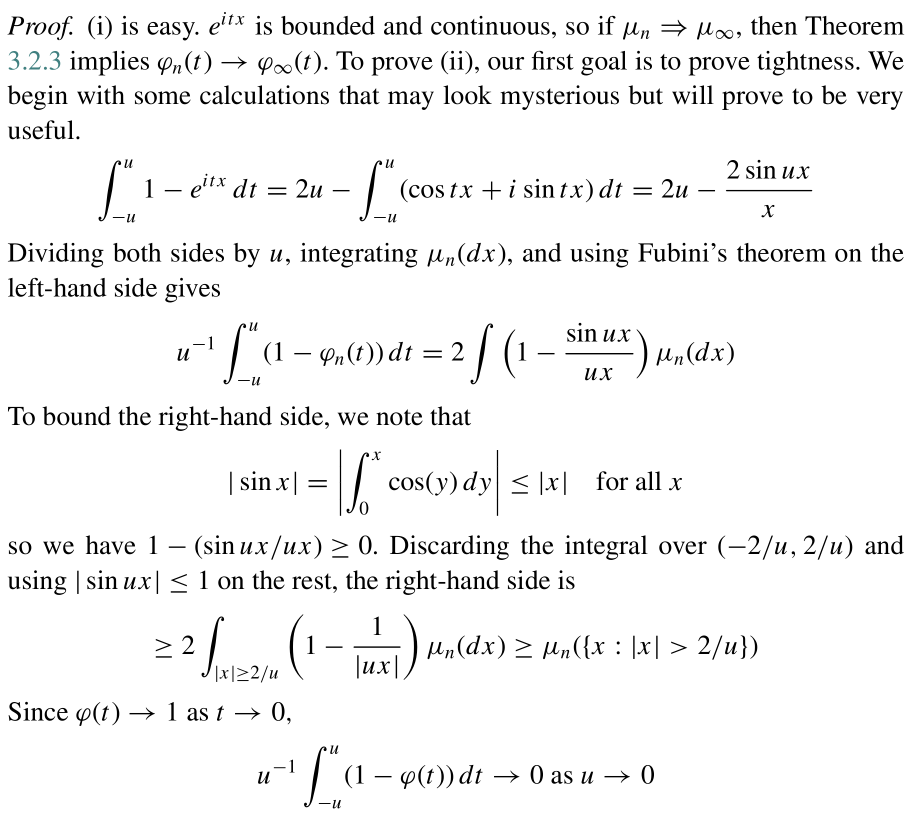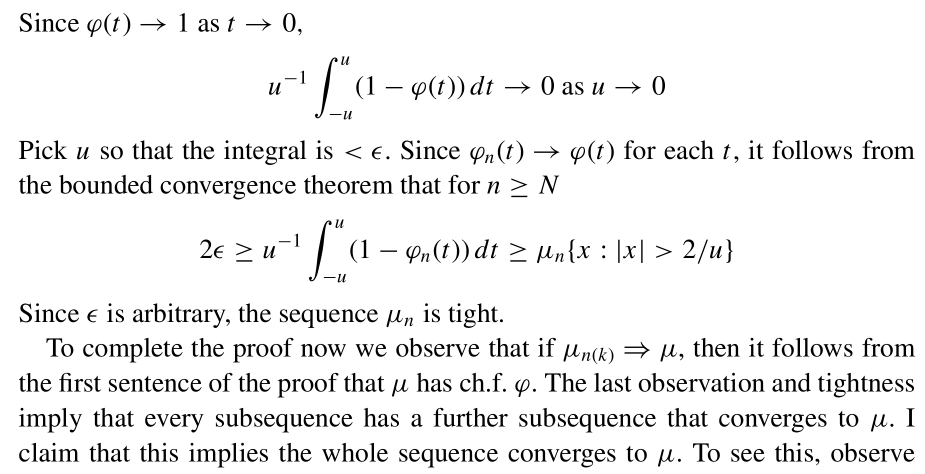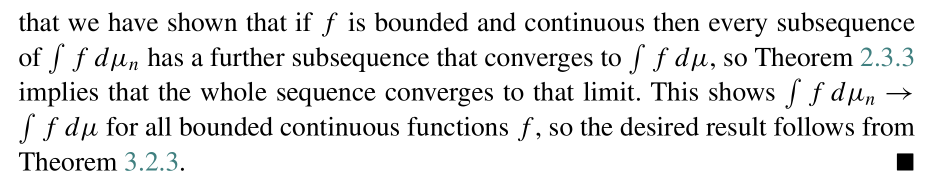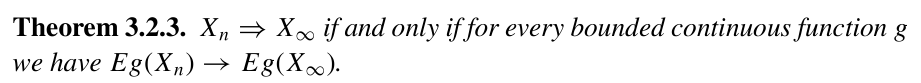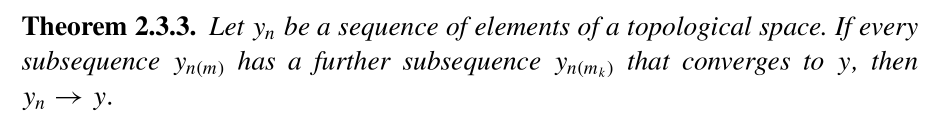展开全文• 概率论中常见分布的数学期望、方差与特征函数推导 （一）离散型分布 #1.单点分布 #2.两点分布 #3.二项分布 #4.泊松分布 #5.超几何分布 #6.几何分布 #7.负二项分布 1.单点分布 随机变量的取值，X=a(常数) 分布律：P(X...统计学
• 非常好的 概率论中 关于特征函数 和 矩母函数的介绍；
• 特征函数的性质知：已知\varphi_{X_i}(t)=（1-\frac{it}{\lambda}）^{-1},由特征函数的性质知：已知φXi​​(t)=（1−λit​）−1,由特征函数的性质知： φX(t)=[φXi(t)]n=（1−itλ）−n\varphi_X(t)=[\varphi_{...
• 生成函数 生成函数我理解是一种为了方便求解特定的某些问题所可以套用的模型。例如我们要求解骰子取值的期望、方差、或是两个骰子点数和的概率等等这些问题，可以用同一个生成函数解决。...特征函数...生成函数
• Z=X+Y的分布密度函数fz(z)为X,Y的密度函数的卷积 接下来我们再看个问题，概率论中定义g(u) = E[e^juX]为随机变量X的特征函数（有很多方便之处，所以数学家才定义了这个），我们展开看看： 即：g(u) = fx(x) * e^jux...概率密度函数 卷积 傅里叶变换
• 本文档以图片形式介绍概率论中一个重要的概念，特征函数。包括特征函数的类型，基本定义以及对应的性质，常见几种分布的特征函数推导过程。
• 概率论中，特征函数的益处体现在： 任意分布与它的特征函数一一对应； 两个独立随机变量之和的特征函数就是它们二者特征函数的积； 特征函数在零点附近收敛 == 分布函数弱收敛(Levi continuous theroem)，要...
• 在[概率论](httpszh.wikipedia.orgwiki%E6%A6%82%E7%8E%87%E8%AE%BA 概率论)中，任何[随机变量](httpszh.wikipedia.orgwiki%E9%9A%8F%E6%9C%BA%E5%8F%98%E9%87%8F 随机变量)的特征函数完全定义了它的[概率分布]...
• 概率论中，任何随机变量的特征函数（缩写：ch.f，复数形式：ch.f’s）完全定义了它的概率分布。 特征函数能够唯一确定随机变量的概率分布，如果随机变量的概率密度函数f(x)存在，特征函数相当 f(x)的傅里叶变换。 ...傅里叶变换
• 特征函数可以算是概率论中的一个工具，其本质是分布的一个傅里叶变换。随机过程
• 概率论第四章复习。 文章目录数学期望含义离散型定义举例Bernoulli分布E1A=P(A)E_{1_A}=P(A)E1A​​=P(A).Possion分布非负随机变量的期望几何分布连续型非负随机变量的期望指数分布柯西分布一般情形函数的期望数学...
• 随机过程及应用-特征函数（Stocbastic Processes With Its Applications ）： || **1....为简化计算量，将傅里叶变换引入并用于分布函数（概率分布、分布律），就产生了* 特征函数 *，经概率论乃至随
• 随机变量在概率空间中遵循不同类型的分布，这决定了它们的特征并有助于预测。 本文内容列表： 引言 高斯/正态分布（Gaussian/Normal Distribution） 二项分布(Binomial Distribution) 伯努利分布(Bernoulli ...机器学习 概率分布 统计学
• 文章目录概率论之随机变量的数字特征一、数学期望1.1、定义1.2、常用分布数学期望及方差1.3、一维随机变量函数的数学期望1.4、数学期望的性质二、中位数三、方差3.1、定义3.2、方差的计算3.3、方差的性质四、矩、...
• 描述量是从分布中提取出的一个数值，用来表示分布的某个特征。之前使用了两个描述量，即期望和方差。在期望和方差之外，还有其它的描述量吗? 　斜度 　值得思考的是，期望和方差足以用来描述一个分布吗?如果答案是...
• 本书论述了以测度论为基础的概率论的最基本的概念、方法和理论，介绍了在概率论中起重要作用的随机变量特征函数的主要内容等数学
• 概率论与数理统计(4):随机变量的数字特征 文章目录概率论与数理统计(4):随机变量的数字特征一.数学期望引例：频率与概率1.离散型随机变量①定义②常见期望证明：两点分布二项分布泊松分布③离散型随机变量函数的数学...数学
• 数学期望期望：离散型：E(X) = X1*p(X1） + X2*p(X2） + …… + Xn*p(Xn) = X1*f1(X1） + X2*f2(X2） + …… + Xn*fn(Xn)连续... u1,u2,…,un,…依次用加号连接起来的函数.数项级数的简称.如：u1+u2+…+un+…,简写为∑u数学期望 绝对收敛
• 第一章 概率论的基本概念 0x110x110x11 随机试验 一、随机现象 ① 确定性现象 定义：在一定条件下必然发生的现象称为确定性现象. 确定性现象的特征：条件完全决定结果 ② 随机现象 定义：在一定条件下可能出现也可能...
• 多维随机变量的特征数 数学期望 二维随机变量的数学期望 若二维随机变量 (X,Y)(X,Y)(X,Y) 的分布用联合发布列或联合密度函数表示，则 Z=g(X,Y)Z=g(X,Y)Z=g(X,Y) 的数学期望为 E(Z)={∑i∑jg(xi,yj)P(X=xi,Y=yj)离散......